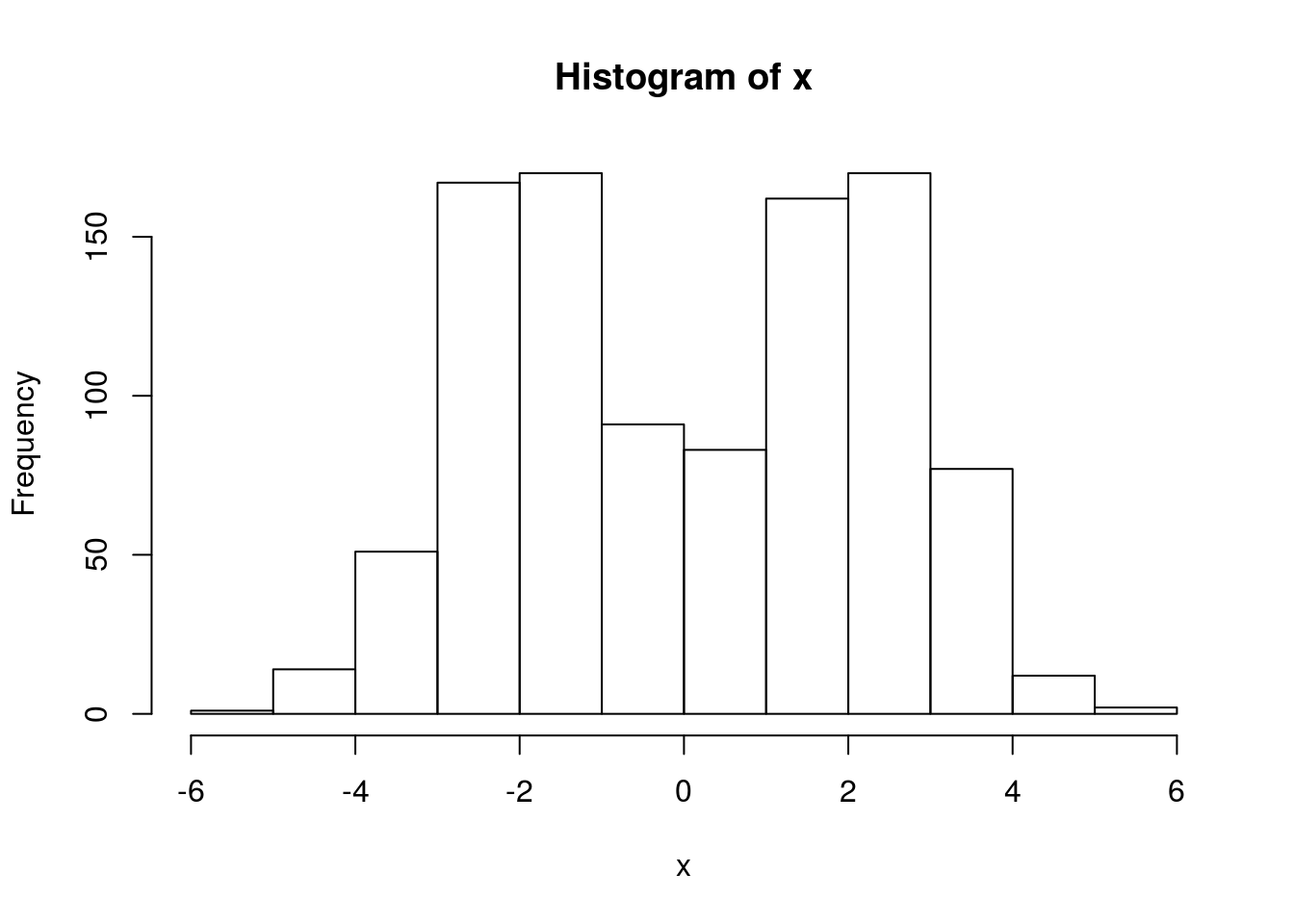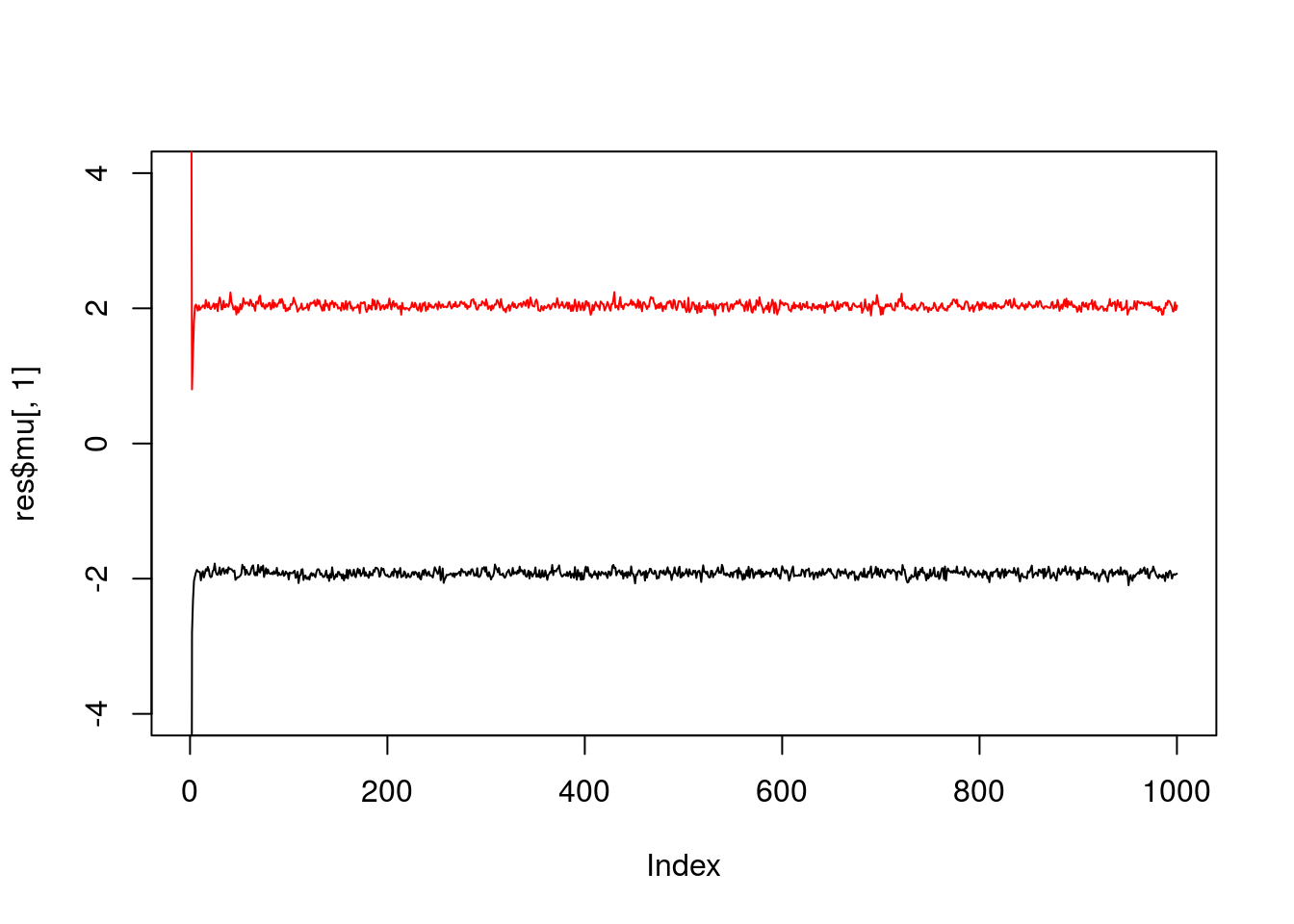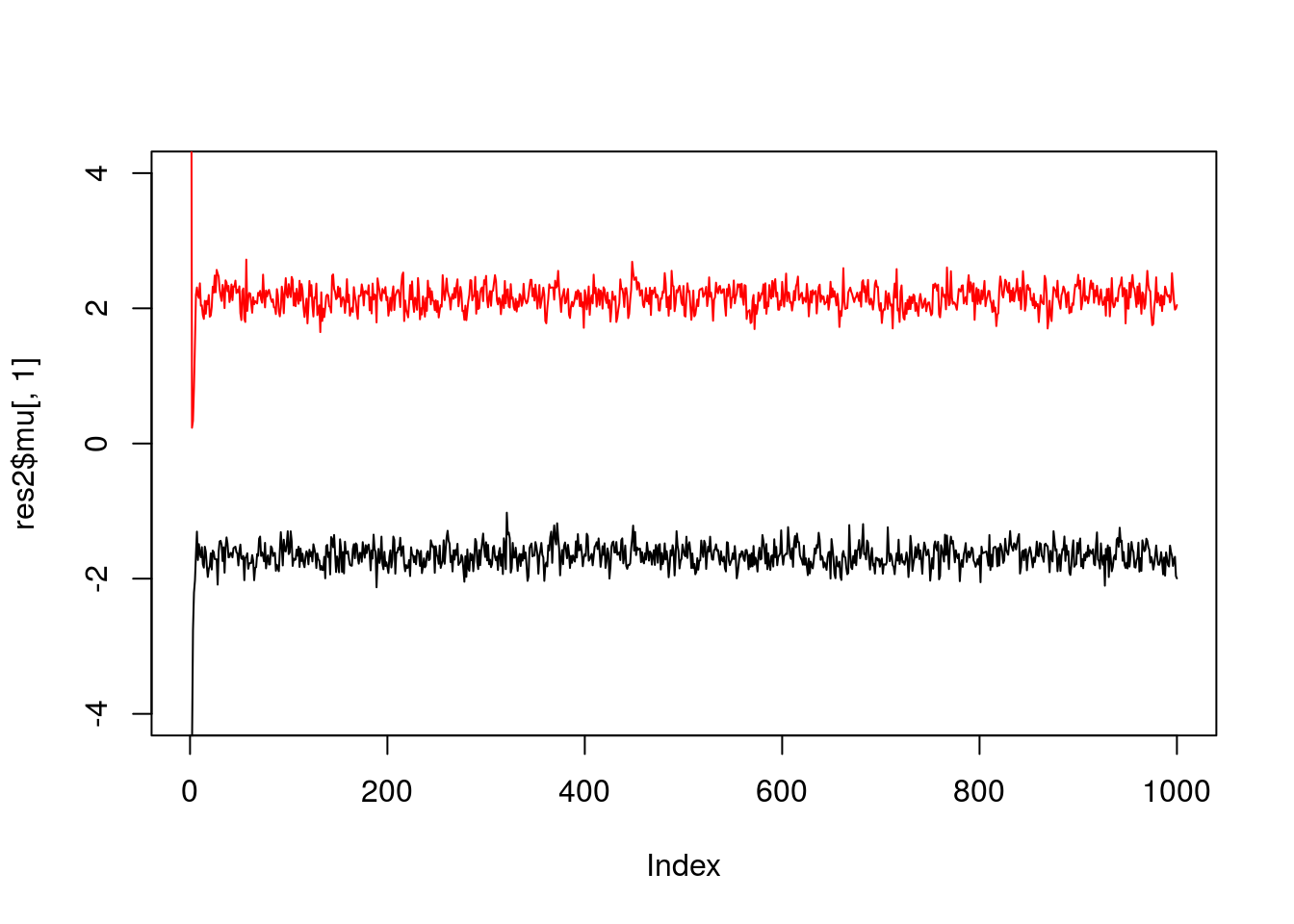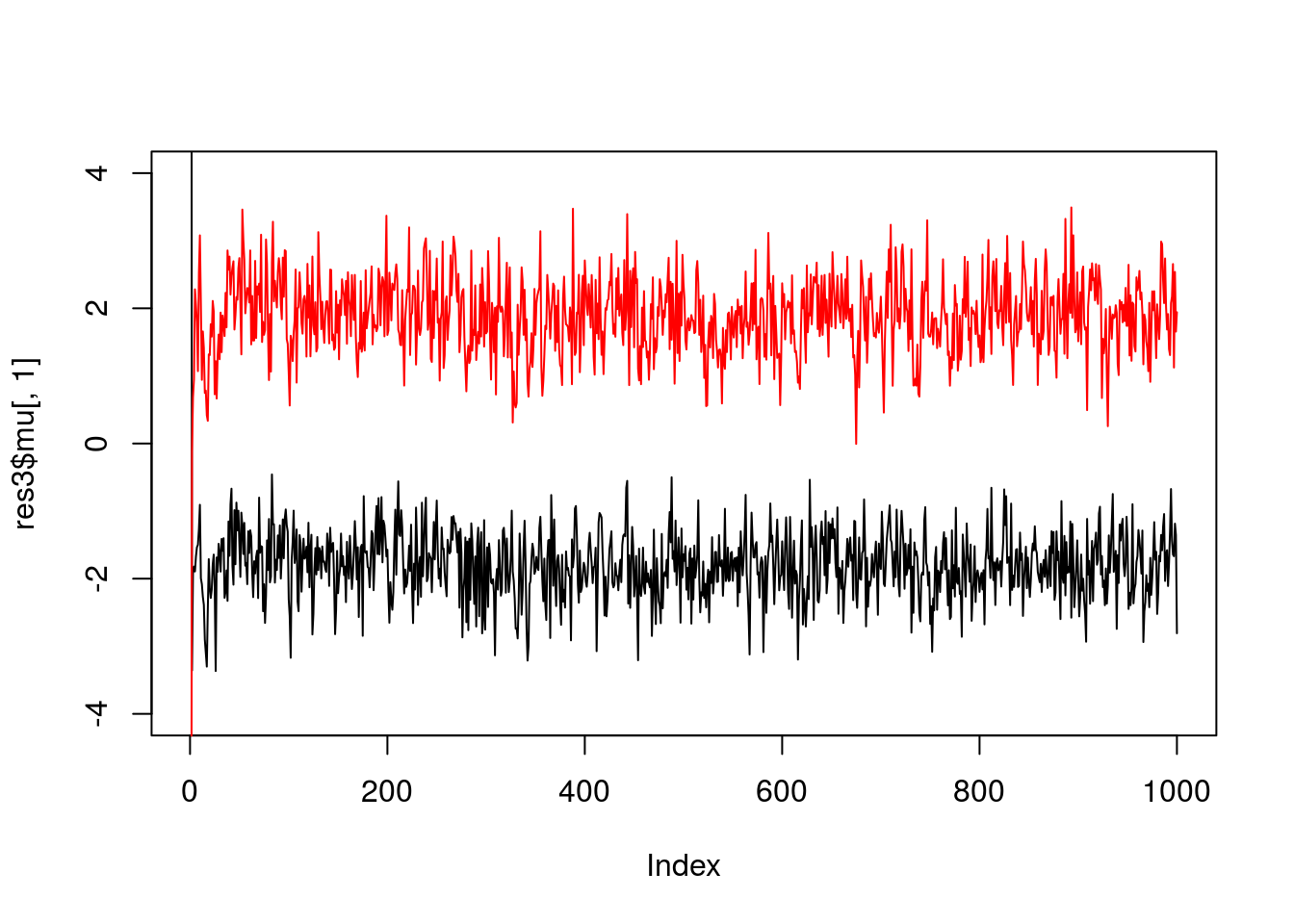Last updated: 2017-03-06

Code version: c7339fc

# Pre-requisites

Know what a Gibbs sampler is, and a mixture model is, and be familiar with Bayesian inference for a normal mean and for the two class problem.

# Overview

We consider using Gibbs sampling to perform inference for a normal mixture model, $X_1,\dots,X_n \sim f(\cdot)$ where $f(\cdot) = \sum_{k=1}^K \pi_k N(\cdot; \mu_k,1).$ Here $$\pi_1,\dots,\pi_K$$ are non-negative and sum to 1, and $$N(\cdot;\mu,\sigma^2)$$ denotes the density of the $$N(\mu,\sigma^2)$$ distribution.

Recall the latent variable representation of this model: $\Pr(Z_j = k) = \pi_k$ $X_j | Z_j = k \sim N(\mu_k,1)$

To illustrate, let’s simulate data from this model:

set.seed(33)

# generate from mixture of normals
#' @param n number of samples
#' @param pi mixture proportions
#' @param mu mixture means
#' @param s mixture standard deviations
rmix = function(n,pi,mu,s){
z = sample(1:length(pi),prob=pi,size=n,replace=TRUE)
x = rnorm(n,mu[z],s[z])
return(x)
}
x = rmix(n=1000,pi=c(0.5,0.5),mu=c(-2,2),s=c(1,1))
hist(x)# Gibbs sampler

Suppose we want to inference for the parameters $$\mu,\pi$$. That is, we want to sample from $$p(\mu,\pi | x)$$. We can use a Gibbs sampler. However, to do this we have to augment the space to sample from $$p(z,\mu,\pi | x)$$, not only $$p(\mu,\pi | x)$$.

Here is the algorithm in outline:

• sample $$\mu$$ from $$\mu | x, z, \pi$$
• sample $$\pi$$ from $$\pi | x, z, \mu$$
• sample $$z$$ from $$z | x, \pi, \mu$$

The point here is that all of these conditionals are easy to sample from.

# Code

  normalize = function(x){return(x/sum(x))}

#' @param x an n vector of data
#' @param pi a k vector
#' @param mu a k vector
sample_z = function(x,pi,mu){
dmat = outer(mu,x,"-") # k by n matrix, d_kj =(mu_k - x_j)
p.z.given.x = as.vector(pi) * dnorm(dmat,0,1)
p.z.given.x = apply(p.z.given.x,2,normalize) # normalize columns
z = rep(0, length(x))
for(i in 1:length(z)){
z[i] = sample(1:length(pi), size=1,prob=p.z.given.x[,i],replace=TRUE)
}
return(z)
}

#' @param z an n vector of cluster allocations (1...k)
#' @param k the number of clusters
sample_pi = function(z,k){
counts = colSums(outer(z,1:k,FUN="=="))
pi = gtools::rdirichlet(1,counts+1)
return(pi)
}

#' @param x an n vector of data
#' @param z an n vector of cluster allocations
#' @param k the number o clusters
#' @param prior.mean the prior mean for mu
#' @param prior.prec the prior precision for mu
sample_mu = function(x, z, k, prior){
df = data.frame(x=x,z=z)
mu = rep(0,k)
for(i in 1:k){
sample.size = sum(z==i)
sample.mean = ifelse(sample.size==0,0,mean(x[z==i]))

post.prec = sample.size+prior$prec post.mean = (prior$mean * prior$prec + sample.mean * sample.size)/post.prec mu[i] = rnorm(1,post.mean,sqrt(1/post.prec)) } return(mu) } gibbs = function(x,k,niter =1000,muprior = list(mean=0,prec=0.1)){ pi = rep(1/k,k) # initialize mu = rnorm(k,0,10) z = sample_z(x,pi,mu) res = list(mu=matrix(nrow=niter, ncol=k), pi = matrix(nrow=niter,ncol=k), z = matrix(nrow=niter, ncol=length(x))) res$mu[1,]=mu
res$pi[1,]=pi res$z[1,]=z
for(i in 2:niter){
pi = sample_pi(z,k)
mu = sample_mu(x,z,k,muprior)
z = sample_z(x,pi,mu)
res$mu[i,] = mu res$pi[i,] = pi
res$z[i,] = z } return(res) } Try the Gibbs sampler on the data simulated above. We see it quickly moves to a part of the space where the mean parameters are near their true values (-2,2).  res = gibbs(x,2) plot(res$mu[,1],ylim=c(-4,4),type="l")
lines(res$mu[,2],col=2)If we simulate data with fewer observations we should see more uncertainty  x = rmix(100,c(0.5,0.5),c(-2,2),c(1,1)) res2 = gibbs(x,2) plot(res2$mu[,1],ylim=c(-4,4),type="l")
lines(res2$mu[,2],col=2)And fewer observations still…  x = rmix(10,c(0.5,0.5),c(-2,2),c(1,1)) res3 = gibbs(x,2) plot(res3$mu[,1],ylim=c(-4,4),type="l")
lines(res3$mu[,2],col=2)And we can get credible intervals (CI) from these samples (discard the first few samples as “burn-in”). For example, to get 90% posterior CIs for the mean parameters:  quantile(res3$mu[-(1:10),1],c(0.05,0.95))
       5%       95%
-2.644896 -1.004009 
  quantile(res3\$mu[-(1:10),2],c(0.05,0.95))
       5%       95%
0.9400428 2.7773584 

## Session information

sessionInfo()
R version 3.3.2 (2016-10-31)
Platform: x86_64-pc-linux-gnu (64-bit)
Running under: Ubuntu 14.04.5 LTS

locale:
 LC_CTYPE=en_US.UTF-8       LC_NUMERIC=C
 LC_TIME=en_US.UTF-8        LC_COLLATE=en_US.UTF-8
 LC_MONETARY=en_US.UTF-8    LC_MESSAGES=en_US.UTF-8
 LC_PAPER=en_US.UTF-8       LC_NAME=C
 LC_MEASUREMENT=en_US.UTF-8 LC_IDENTIFICATION=C

attached base packages:
 stats     graphics  grDevices utils     datasets  methods   base

other attached packages:
 workflowr_0.4.0    rmarkdown_1.3.9004

loaded via a namespace (and not attached):
 backports_1.0.5 magrittr_1.5    rprojroot_1.2   htmltools_0.3.5
 tools_3.3.2     yaml_2.1.14     Rcpp_0.12.9     stringi_1.1.2
 knitr_1.15.1    git2r_0.18.0    stringr_1.2.0   digest_0.6.12
 gtools_3.5.0    evaluate_0.10  

This site was created with R Markdown